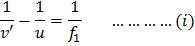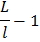# Two thin lenses of focal lengthsandare placed in contact with each other. The focal length of the combination is a)b)c)d)## Question ID - 152415 :- Two thin lenses of focal lengthsandare placed in contact with each other. The focal length of the combination is a)b)c)d)3537

 (c) Whenandare focal lengths of lenses combined together, image formation takes place as follows From lens formulaAdding Eqs. (i) and (ii), we getIf this lens is replaced by a single lens, then focal length of combination isIn an astronomical telescope in normal adjustment, a straight black line of lengthis drawn on the objective lens. The eyepiece forms a real image of this line. The length of this image is. The magnification of the telescope is a)b)c)d)